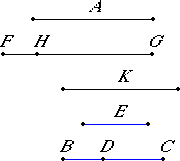# Proposition 87

To find the third apotome.

Set out a rational straight line A. Set out three numbers E, BC, and CD which do not have to one another the ratio which a square number has to a square number, but let CB have to BD the ratio which a square number has to a square number.X.6,Cor.

Let it be contrived that E is to BC as the square on A is to the square on FG, and BC is to CD as the square on FG is to the square on GH.

X.6

Since E is to BC as the square on A is to the square on FG, therefore the square on A is commensurable with the square on FG.

But the square on A is rational, therefore the square on FG is also rational, therefore FG is rational.

X.9

Since E does not have to BC the ratio which a square number has to a square number, therefore neither has the square on A to the square on FG the ratio which 3 square number has to a square number. Therefore A is incommensurable in length with FG.

X.6

Again, since BC is to CD as the square on FG is to the square on GH, therefore the square on FG is commensurable with the square on GH.

But the square on FG is rational, therefore the square on GH is also rational, therefore GH is rational.

X.9

Since BC does not have to CD the ratio which a square number has to a square number, therefore neither has the square on FG to the square on GH the ratio which a square number has to a square number. Therefore FG is incommensurable in length with GH.

X.73

And both are rational, therefore FG and GH are rational straight lines commensurable in square only. Therefore FH is an apotome.

I say next that it is also a third apotome.

V.22

Since E is to BC as the square on A is to the square on FG, and BC is to CD as the square on FG is to the square on HG, therefore, ex aequali, E is to CD as the square on A is to the square on HG.

X.9

But E does not have to CD the ratio which a square number has to a square number, therefore neither has the square on A to the square on GH the ratio which a square number has to a square number. Therefore A is incommensurable in length with GH.

Therefore neither of the straight lines FG nor GH is commensurable in length with the rational straight line A set out.

Now let the square on K be that by which the square on FG is greater than the square on GH.

V.19,Cor.

Since BC is to CD as the square on FG is to the square on GH, therefore, in conversion, BC is to BD as the square on FG is to the square on K.

But BC has to BD the ratio which a square number has to a square number, therefore the square on FG also has to the square on K the ratio which a square number has to a square number.

X.9

Therefore FG is commensurable in length with K, and the square on FG is greater than the square on GH by the square on a straight line commensurable with FG.

X.Def.III.3

And neither of the straight lines FG nor GH is commensurable in length with the rational straight line A set out, therefore FH is a third apotome.

Therefore the third apotome FH has been found.

Q.E.F.

## Guide

This proposition is not used in the rest of the Elements.